Project in progress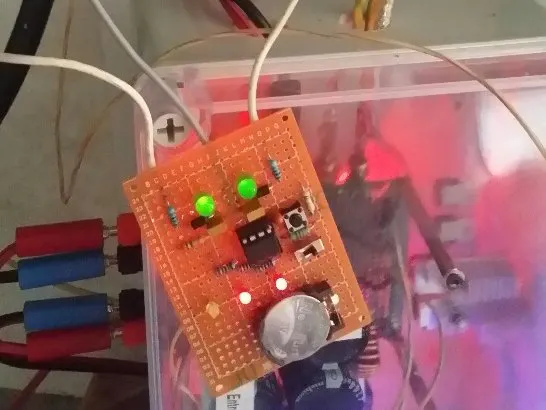# Arduino / ATtiny85 Delta AC 3-Phase Checker © GPL3+

This is a very simple way to control a 3-phase AC installation in a delta configuration.

• 3,263 views
• 11 respects

## Necessary tools and machinesSoldering iron (generic)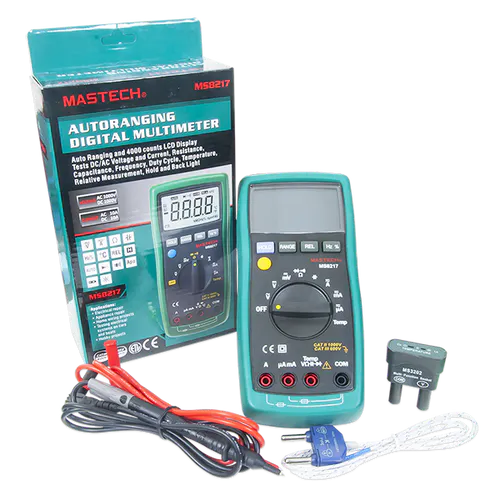Digilent Mastech MS8217 Autorange Digital Multimeter

## Apps and online services

For those who have a wind turbine in their garden, one of the worst thing that can happen is to see the propeller smoothy turn and turn and suddenly stop for a very short time and then turn again, without apparently any reason to stop. What has happened ????

May be it is the 3-wires cable that has a short circuit.... How to see ? With a voltmeter of course, you can verify... but most of time you will only measure that everything is right. Because of course the short-circuit is very brief, very short.

Here is a solution : when installed, if everything is all right the 2 red leds are off. But as soon as no voltage is detected between 2 of the 3 wires (or the 3 wires) one led will flash which indicates which wire has a problem :

Red led 1 if there is a problem between volt1 and volt3, red led 2 if there is a problem between volt2 and volt3, both red led indicates a problem between volt2 and volt3, or, that there is no more wind....

R is set to limit current : 4.7K will make a current of 10mA for a voltage of 47V, and 20mA for 94V.

D is a rectifier diode, like 1N4001 or whatever you want.

LED (green led ) acts as a zener to limit the input voltage to the analog input of the Arduino. I use a years 90 green led that has its working voltage arround 2V. You can use whatever LED you wish, at the condition its working voltage is under 2.5V if used with the ATtiny85.

What does the code perform ?

It continuously reads the volt1 and volt2 voltage for 100 cycles. Don't forget it is AC voltage, so a little time is needed to insure to get several periods of the voltage. If after this time the value is too low, then it stops the measures and the right red led is flashing, until a reset. At the same time a comparison is done between volt1 and volt2, if measured values are too near, both red leds are flashing.

The code is proposed for Arduino, and ATtiny85.

The advantage of the ATtiny85 is very small, so it is possible to build a very small size device that can operate under 3V with a CR2032 cell. Thanks to the sleeping method in the code that will insure the cell will operate for years !

## Code

##### Arduino uno code for the 3-phases checkerC/C++
Arduino uno code for the 3-phases checker
```/* 3 phases checker in triangle configuration
*
*  For Arduino Uno
*
*

RESET  => Arduino Uno push-button
volt1 = A3  => 1st wire
volt2 = A2  => 2nd wire
volt3 = GND => 3rd wire
LED1 = 8   => 1st wire LED
LED2 = 9   => 2nd wire LED

*/

bool v1cut = false, v2cut = false;

void setup() {
pinMode(8, OUTPUT);
pinMode(9, OUTPUT);
}

void flasher(byte p) {
digitalWrite(p, HIGH);
delay(5);
digitalWrite(p, LOW);
}

void loop() {
unsigned long volt1, volt2;
byte nbre_cc;
byte a=0;

while( v1cut == false && v2cut == false ) {
volt1=0; volt2=0;
nbre_cc=0;
a++;
if( a%10 == 0 ) flasher(0);
for( byte i=0; i<100; i++ ) {
delayMicroseconds(10);
delayMicroseconds(10);
volt1 += v1; volt2 += v2;
if( v1-v2 < 20 || v2-v1 < 20 ) nbre_cc++;
delay(1);
}

if( nbre_cc > 50 ) { v1cut = true; v2cut = true; }
if( volt1 < 1000 ) v1cut = true;
if( volt2 < 1000 ) v2cut = true;
}   // end of while

if( v1cut == true ) flasher(2);
if( v2cut == true ) flasher(1);
}   // end of loop
```
##### ATtiny85 3-phases checker codeC/C++
ATtiny85 3-phases checker code
```/* 3 phases checker in triangle configuration
*
*  For ATiny85
*
*
+-------+
RESET  <= PB5  1|*      |8  VCC
input1 <= A3 = PB3  2|       |7  PB2 => LED1
input2 <= A2 = PB4  3|       |6  PB1 => LED2
input3 <= GND  4|       |5  PB0 => startup LED
+-------+
connection :

RESET in PB5 = pin1  => push-button to GND // 10K to Vcc
volt1 in PB3 = pin2  => 1st wire
volt2 in PB4 = pin3  => 2nd wire
GND = pin4  => 3rd wire
LED1 in PB2 = pin7  => 1st wire
LED2 in PB1 = pin6  => 2nd wire
startup LED PB0 = pin5  => flash after each reset push

*/

#include <avr/sleep.h>
#include <EEPROM.h>

#ifndef cbi
#define cbi(sfr, bit) (_SFR_BYTE(sfr) &= ~_BV(bit))
#endif
#ifndef sbi
#define sbi(sfr, bit) (_SFR_BYTE(sfr) |= _BV(bit))
#endif

bool eveil = true;                      // WD flag
bool v1cut = false, v2cut = false;

void setup() {

pinMode(0, INPUT);
pinMode(3, INPUT);
pinMode(4, INPUT);
pinMode(2, INPUT);
pinMode(1, INPUT);

// the watchdog sets the interval delay :
// 0=16ms, 1=32ms,2=64ms, 3=128ms, 4=250ms, 5=500ms, 6=1s, 7=2s, 8=4s, 9=8s
setup_watchdog(6);
set_sleep_mode(SLEEP_MODE_PWR_DOWN);  // sleep mode

for( byte i=0; i<5; i++ ) {
flasher(0);
delay(100);
}
}

void flasher(byte p) {
pinMode(p, OUTPUT);
digitalWrite(p, HIGH);
delay(5);
digitalWrite(p, LOW);
pinMode(p, INPUT);
}

void loop() {

unsigned long volt1, volt2;
byte nbre_cc;
byte a=0;

while( v1cut == false && v2cut == false ) {
volt1=0; volt2=0;
nbre_cc=0;
a++;
if( a%10 == 0 ) flasher(0);
for( byte i=0; i<100; i++ ) {
delayMicroseconds(10);
delayMicroseconds(10);
volt1 += v1; volt2 += v2;
if( v1-v2 < 20 || v2-v1 < 20 ) nbre_cc++;
delay(1);
}

if( nbre_cc > 50 ) { v1cut = true; v2cut = true; }
if( volt1 < 1000 ) v1cut = true;
if( volt2 < 1000 ) v2cut = true;
}   // end of while

if( eveil == true ) {
eveil = false;
if( v1cut == true ) flasher(2);
if( v2cut == true ) flasher(1);
system_sleep();           // go to sleep for 8 secondes
}
}   // end of loop

// set system into the sleep state - system wakes up when wtchdog is timed out
void system_sleep() {
EEPROM.write(0, v1cut);
EEPROM.write(1, v2cut);
sleep_enable();
sleep_mode();               // System actually sleeps here
sleep_disable();            // System continues execution here when watchdog timed out
}

void setup_watchdog( byte tempo ) {
byte val;
val = tempo & 7;
if (tempo > 7) val |= (1<<5);
val |= (1<<WDCE);
MCUSR &= ~(1<<WDRF);                  // start timed sequence
WDTCR |= (1<<WDCE) | (1<<WDE);        // set new watchdog timeout value
WDTCR = val;
WDTCR |= _BV(WDIE);
}

// Watchdog Interrupt Service / is executed when watchdog timed out
ISR(WDT_vect) { eveil = true; }        // set global flag
```

## Custom parts and enclosures

Here is an example of what can look like an ATtiny85 3-phases checker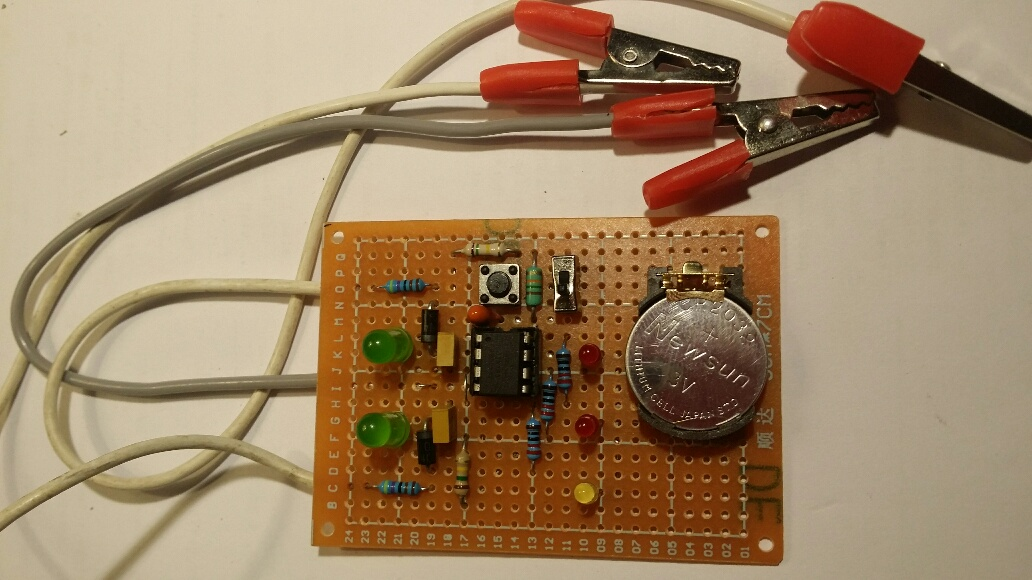## Schematics

Arduino diagram for 3-phases checker.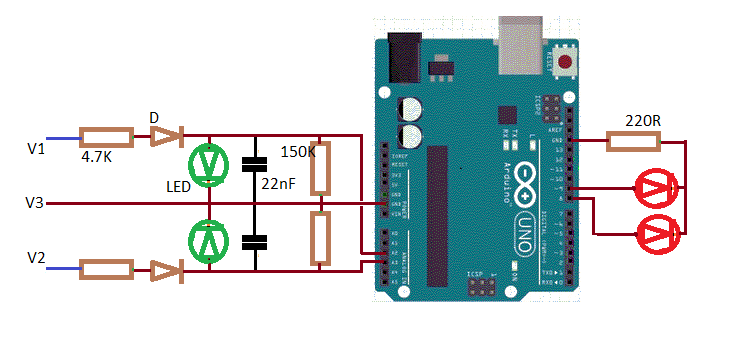ATtiny85 3-phases checker diagram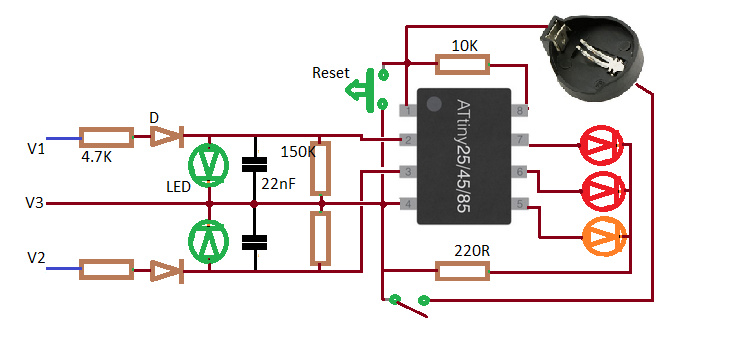#### Arduino / ESP8266 RS485 MODBUS Anemometer

Project in progress by philippedc

• 7,232 views
• 12 respects

#### ATtiny85 Pulse Oximeter and Photoplethysmograph

Project in progress by Jeff Magee

• 9,504 views
• 69 respects

#### 3D-Printed Nanoleaf ATtiny85

Project tutorial by Arnov Sharma

• 3,743 views
• 15 respects

#### Hacking Qualcomm (Quick Charge) QC 2.0/3.0 With ATtiny85

Project tutorial by Shahariar

• 15,388 views
• 47 respects

#### Arduino and AC Devices - Automatic Lights

Project tutorial by Ahmed Hamdy

• 28,780 views